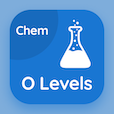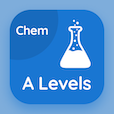SAT Prep Courses

SAT Chemistry MCQ Questions

SAT Chemistry MCQs - Chapters

Learn Mole Concept Multiple Choice Questions and Answers (MCQs), Mole Concept quiz answers PDF to study chemistry course test 1 for online classes. Molar Mass MCQs, Mole Concept Trivia Questions and answers for placement and to prepare for job interview. Mole Concept Book PDF: molar mass, empirical formula career test to learn free online courses.

"The sum of atomic mass of all the atoms present in one molecule of a molecular compound is" Multiple Choice Questions (MCQ Quiz): mole concept App APK with molecular mass, ionic mass, formula unit, and atomic number choices to enroll in online colleges. Practice molar mass quiz questions for jobs' assessment test and online courses for jobs' assessment test and online courses for employment assessment test.

## MCQs on Mole Concept Quiz

MCQ: The sum of atomic mass of all the atoms present in one molecule of a molecular compound is

Ionic Mass
Molecular Mass
Formula unit
Atomic number

MCQ: In combustion analysis, % of hydrogen is calculated from

CH
CO
H2O
CO2

MCQ: In combustion analysis, % of hydrogen is calculated from

C6 H6
CH
CHO
CO2

MCQ: The formula mass of an ionic compound is called

Gram formula
Simple formula
Empirical formula
Molecular formula

MCQ: The molecular mass of H2O is

18 grams
18 amu
1.8 grams
180 amu